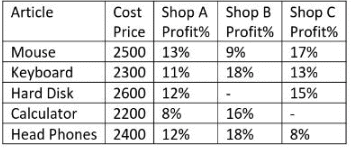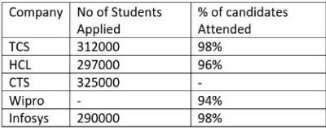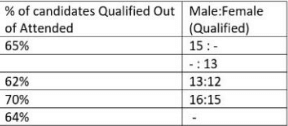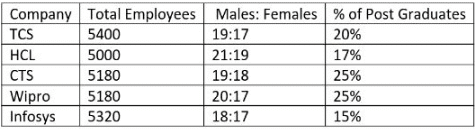# Exam Based Questions - MCQ 5

## 20 Questions MCQ Test Quantitative Aptitude for Competitive Examinations | Exam Based Questions - MCQ 5

Description
Attempt Exam Based Questions - MCQ 5 | 20 questions in 15 minutes | Mock test for Quant preparation | Free important questions MCQ to study Quantitative Aptitude for Competitive Examinations for Quant Exam | Download free PDF with solutions
QUESTION: 1

Solution:

X = 7/5, -6/10
Y = -3/5 , -7/2.

QUESTION: 2

Solution:

X = 7/2, 8/2
Y = 8/2, 9/2

QUESTION: 3

### I. √2X² + 8X – 64√2 = 0 II. √2Y² – 27Y + 81√2 = 0

Solution:

X = -82, 8/2
Y = 9/2, 92

QUESTION: 4

I. X² + 25X + 156 = 0

II. Y² + 29Y + 210 = 0

Solution:

X= -12,- 13
Y = -14,- 15

QUESTION: 5

I. 3X + 4Y = 64

II. 2X + 3Y = 56

Solution:

X = -32, Y = 40

QUESTION: 6

Study the given table and answer the following questionsQ.

If the difference between the Profits made by Shop A and Shop B on Mouse is equal to the Profit earned by Shop C on Calculator then his Profit percent is?

Solution:

2500*(13-9)/100 = Rs 100
2300 = 2200(100+x)/100
X = 50/11%

QUESTION: 7Q.

What is the average profit made Shop A, B and C on Mouse?

Solution:

25*13 = 325

QUESTION: 8Q.

Shop A has made Maximum Profit on which article?

Solution:

Checking by observation
Mouse : 25*13 = 325
Hard Disk: 26*12 = 312

QUESTION: 9Q.

If the total profit made by Shop A, B and C on Hard Disk is Rs.988 then what is the profit of B on Hard Disk?

Solution:

26*(12+x+15) = 988
X = 11%
Profit = 26*11 = 286

QUESTION: 10Q.

Solution:

23*(11+18+13) – 24*(12+18+8)
966 – 912 = 54

QUESTION: 11

Direction (Q. 11-15) Study the given table and answer the following questions.Q.

In Infosys, if the number of Female candidates qualified was 87,808 then what is the respective ratio of the number of Qualified Male candidates to the number of Qualified Female candidates in Infosys?

Solution:

290000*98/100*64/100(F/F+M) = 87808
94080F = 87808M
M/F = 15:14

QUESTION: 12Q.

In HCL, if the ratio of the number of students Attended to the number of candidates qualified is 8:5, and the number of Males qualified was 88704 then number of Females qualified is?

Solution:

If the ratio of number of students Attended to number of candidates qualified is 8:5 then percentage of candidates qualified out of attended is 60%
297*96*6*(M/M+13) = 88704
M = 14
Then Females = 297*96*6*13/27 = 82368

QUESTION: 13Q.

If the difference between the number of Male qualified candidates and the number of Female candidates in Wipro is 5922 then no of candidates attended in Wipro is?

Solution:

X*94/100*70/100*(16-15/31) = 5922
X = 279000
No of candidates attended is 279000*94/100 = 262260

QUESTION: 14Q.

If the number of candidates qualified in TCS is 7319 more than the number of candidates qualified in CTS then the number of Male candidates qualified is how much more than the number of Female candidates in CTS?

Solution:

TCS = 312000*98/100*65/100 = 637*312
CTS = 325000*x/100*62/100 =
TCS – CTS = 73190 = 312000* 98/100* 65/100 – 325000* x/100* 62/100
Then x = 95%
CTS (Males – Females) : 325000*95/100*62/100*(13-12/25) = 7657

QUESTION: 15Q.

In TCS females Qualified forms approximately what percent of Qualified Males if no of Males Qualified is 114660?

Solution:

312000*98/100*65/100*(15/15+x) = 114660
X= 11
% = 11/15*100 = 73.33%

QUESTION: 16

Direction (Q. 16-20) Study the given table and answer the following questions.Q.

If the number of Male Post Graduates in CTS is 648 then no of Female Post Graduates form approximately what percentage of the Female employees in the Same Company?

Solution:

CTS : 5180
Males: 5180*19/37 = 2660 Females: 5180*18/37 = 2520
% of Post Graduates: 5180*25/100 = 1295
% = 647/2520= 25.5%

QUESTION: 17Q.

No of Post Graduates Employees in which of the following company is the least?

Solution:

Infosys :5320*15/100 = 798

QUESTION: 18Q.

What is the ratio of the total number of Male employees in TCS and Wipro together to the total number of Female employees in CTS and Wipro together?

Solution:

Males in TCS : 5400*19/36 = 2850 Males in Wipro: 5180*20/37 = 2800
Females in CTS: 5180*18/37 = 2520 Females in Wipro: 5180*17/37 = 2380
(2850+2800) : (2520+2380)
565: 490
113:98

QUESTION: 19Q.

Number of Post Graduate employees in all companies together forms approximately what percent of the total no of Employees in all companies together?

Solution:

TCS: 5400*20/100 = 1080
HCL: 5000*17/100 = 850
CTS: 5180*25/100 = 1295
Wipro: 5180*25/100 = 1295
Infosys: 5320*15/100 = 798
All Post Graduates: 1080+850+1295+1295+798 = 5318
Total Employees: 5400+5000+5180+5180+5320 = 26080
% = 5318/26080 = 20%

QUESTION: 20Q.

If total number Male Post Graduates in Wipro and Infosys together is 1210 and Female Post Graduates in Wipro and Infosys together is 883 and Number of Female Post Graduate in Wipro forms 40% of total Post Graduates in Wipro then difference of Male and Female Graduates in Infosys is?

Solution:

Post Graduate Females in Wipro = 40% of 1295 = 518 then Post Graduate Males in Wipro = 60% of 1295
= 777Use Code STAYHOME200 and get INR 200 additional OFF Use Coupon Code